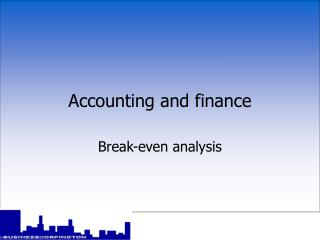DownloadDownload PresentationAccounting and finance

Accounting and finance

Télécharger la présentationAccounting and finance

- - - - - - - - - - - - - - - - - - - - - - - - - - - E N D - - - - - - - - - - - - - - - - - - - - - - - - - - -
Presentation Transcript

1. Accounting and finance Break-even analysis

2. Break-even analysis • break-even analysis provides a simple means of measuring profits and losses at different levels of output • sales revenues and total costs are analysed for each different level of production • analysis is normally done graphically using a break-even chart

3. Drawing a break-even chart £ Sales revenue break- even point at the break-even point, total sales = total cost (i.e. no profit or loss is made) Total costs Break-even sales Fixed costs Break-even output Quantity

4. The margin of safety • the difference between actual output and the break-even output is known as the margin of safety

5. The margin of safety margin of safety Break-even output x units

6. Break-even point • the point at which total costs are covered and no profit or loss is made is called the break-even point • the break-even point is where the total revenue and total cost lines intersect on the chart • this can also be calculated using the formula: BEP = fixed costs contribution per unit

7. Break-even calculation • Assuming that a product has a selling price of £6. Variable costs are £1 per unit and fixed costs are £50,000 per year • Calculate the number of units that a firm must sell in order to break-even • Answer - £50,000 = 1000 units £5

8. Uses of break-even analysis • to calculate the minimum amount of sales required in order to be able to break even • to see how changes in output, selling price or costs will affect profit levels • to calculate the level of output required to reach a certain level of profit • to allow various scenarios (what-if) to be tested out • to aid forecasting and planning

9. Limitations of break-even analysis • it’s accuracy depends upon the accuracy of the data used • forecasting the future is difficult, especially long term • it assumes there is a simple relationship between variable costs and sales • sales income does not necessarily rise in a constant relationship to sales volume • external constraints have to be recognised

10. Summary • the break-even point occurs when total sales revenue matches total costs • the break-even point can be worked out either numerically or graphically • break-even analysis is a very useful tool for businesses to use although it does have some limitations Key concepts: Break-even output Contribution per unit Fixed costs Variable costs Margin of safety